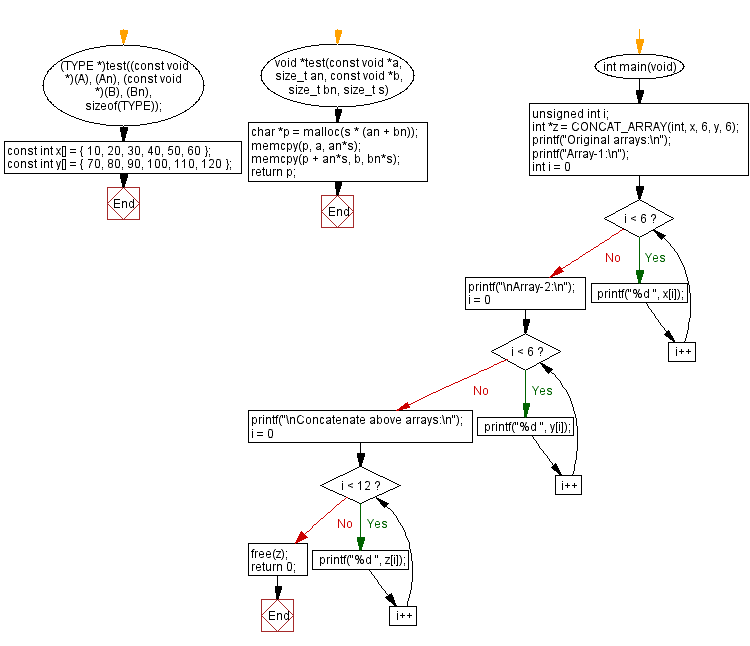﻿ C : Concatenate two arrays# C Exercises: Concatenate two arrays

## C Array: Exercise-107 with Solution

Write a program in C to concatenate two given arrays of integers.
If this is as simple as array1 + array2, so be it.

Sample Data:
({ 10, 20, 30, 40, 50, 60 }, { 70, 80, 90, 100, 110, 120 }) -> "10 20 30 40 50 60 70 80 90 100 110 120"

Sample Solution:

C Code:

``````#include <stdlib.h>
#include <stdio.h>
#include <string.h>

#define CONCAT_ARRAY(TYPE, A, An, B, Bn) \
(TYPE *)test((const void *)(A), (An), (const void *)(B), (Bn), sizeof(TYPE));

void *test(const void *a, size_t an,
const void *b, size_t bn, size_t s)
{
char *p = malloc(s * (an + bn));
memcpy(p, a, an*s);
memcpy(p + an*s, b, bn*s);
return p;
}

const int x[] = { 10, 20, 30, 40, 50, 60 };
const int y[] = { 70, 80, 90, 100, 110, 120 };

int main(void)
{
unsigned int i;

int *z = CONCAT_ARRAY(int, x, 6, y, 6);
printf("Original arrays:\n");
printf("Array-1:\n");
for(int i = 0; i < 6; i++)
printf("%d ", x[i]);
printf("\nArray-2:\n");
for(i = 0; i < 6; i++)
printf("%d ", y[i]);
printf("\nConcatenate above arrays:\n");
for(i = 0; i < 12; i++)
printf("%d ", z[i]);
free(z);
return 0;
}
```
```

Sample Output:

```Original arrays:
Array-1:
10 20 30 40 50 60
Array-2:
70 80 90 100 110 120
Concatenate above arrays:
10 20 30 40 50 60 70 80 90 100 110 120
```

Flowchart:C Programming Code Editor:

Improve this sample solution and post your code through Disqus.

Previous C Programming Exercise: Array, shift all 0 to the end, double the value of next.
Next C Programming Exercise: C Pointer Exercises Home

What is the difficulty level of this exercise?

Test your Programming skills with w3resource's quiz.

﻿